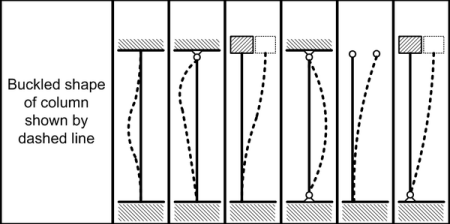This section first calculates the critical slenderness ratio, C cfor the material whose properties are entered, compares this Ccvalue with the slenderness ratio of the column and if the slenderness ratio is greater than Cc , the buckling load is calculated by the Euler formula, whereas if the slenderness ratio is less than Cc, the Johnson formula is used.